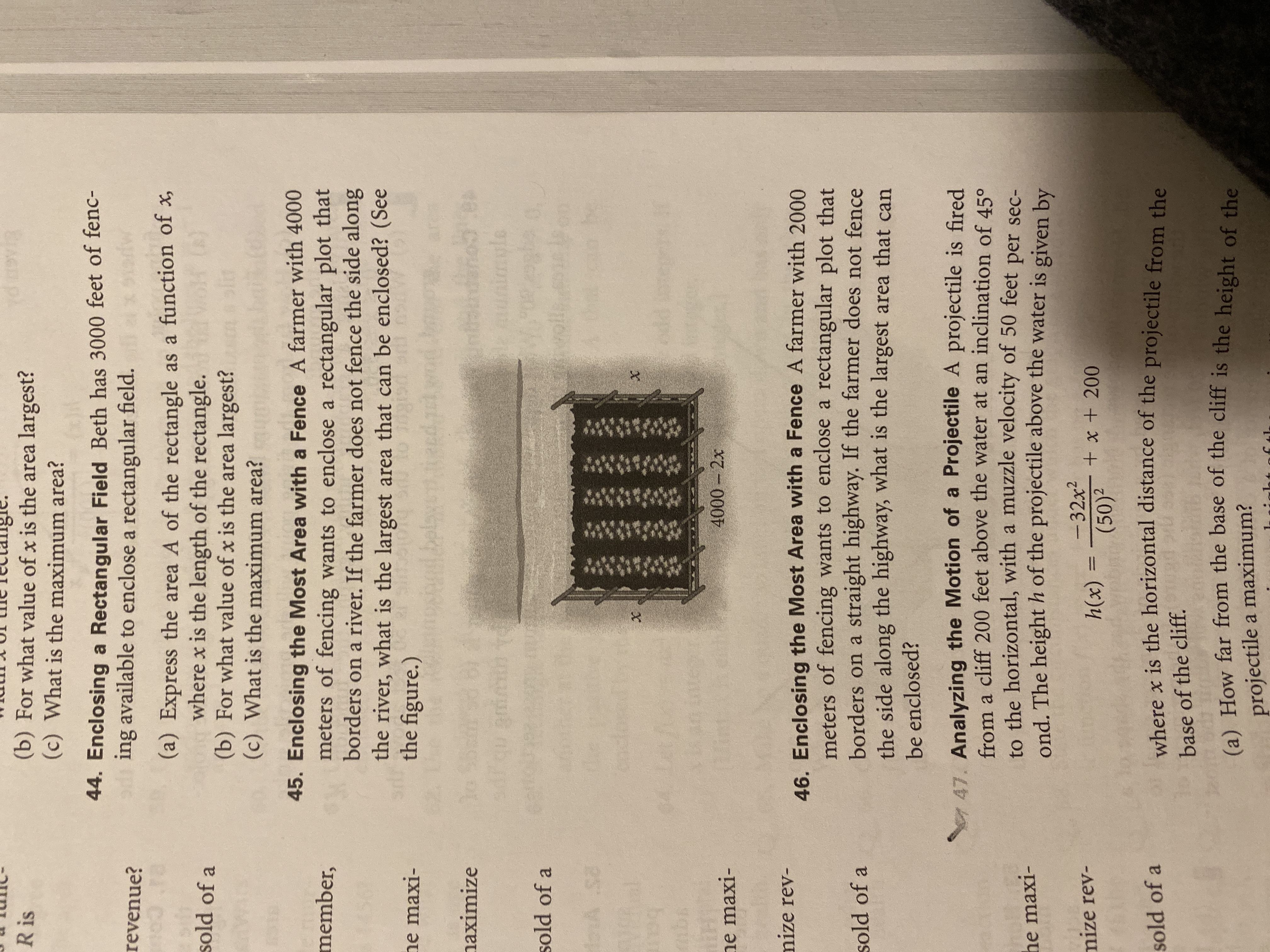# d py (b) For what value of x is the area largest? (c) What is the maximum area? R is 44. Enclosing a Rectangular Field Beth has 3000 feet of fenc- ing available to enclose a rectangular field. revenue? (a) Express the area A of the rectangle as a function of x where x is the length of the rectangle. (b) For what value of x is the area largest? (c) What is the maximum area? sold of a 45. Enclosing the Most Area with a Fence A farmer with 4000 meters of fencing wants to enclose a rectangular plot that borders on a river. If the farmer does not fence the side along member, the river, what is the largest area that can be enclosed? (See the figure.) he maxi- belay lo naximize le 0.5 VIpes sold of a douA S2 X m 4000-2x ne maxi- mize rev- 46. Enclosing the Most Area with a Fence A farmer with 2000 meters of fencing wants to enclose a rectangular plot that borders on a straight highway. If the farmer does not fence the side along the highway, what is the largest area that can sold of a be enclosed? 7 47. Analyzing the Motion of a Projectile A projectile is fired from a cliff 200 feet above the water at an inclination of 45° to the horizontal, with a muzzle velocity of 50 feet per sec- ond. The height h of the projectile above the water is given by he maxi- -32x2 mize rev- + x + 200 h(x) (50)2 ahere x is the horizontal distance of the projectile from the sold of a base of the cliff. (a) How far from the base of the cliff is the height of the projectile a maximum?

Question

I need help with number 46help_outlineImage Transcriptionclosed py (b) For what value of x is the area largest? (c) What is the maximum area? R is 44. Enclosing a Rectangular Field Beth has 3000 feet of fenc- ing available to enclose a rectangular field. revenue? (a) Express the area A of the rectangle as a function of x where x is the length of the rectangle. (b) For what value of x is the area largest? (c) What is the maximum area? sold of a 45. Enclosing the Most Area with a Fence A farmer with 4000 meters of fencing wants to enclose a rectangular plot that borders on a river. If the farmer does not fence the side along member, the river, what is the largest area that can be enclosed? (See the figure.) he maxi- belay lo naximize le 0.5 VIpes sold of a douA S2 X m 4000-2x ne maxi- mize rev- 46. Enclosing the Most Area with a Fence A farmer with 2000 meters of fencing wants to enclose a rectangular plot that borders on a straight highway. If the farmer does not fence the side along the highway, what is the largest area that can sold of a be enclosed? 7 47. Analyzing the Motion of a Projectile A projectile is fired from a cliff 200 feet above the water at an inclination of 45° to the horizontal, with a muzzle velocity of 50 feet per sec- ond. The height h of the projectile above the water is given by he maxi- -32x2 mize rev- + x + 200 h(x) (50)2 ahere x is the horizontal distance of the projectile from the sold of a base of the cliff. (a) How far from the base of the cliff is the height of the projectile a maximum? fullscreen

### Want to see the step-by-step answer?